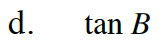Home > CCA2 > Chapter 12 > Lesson 12.1.4 > Problem12-67

12-67.
1.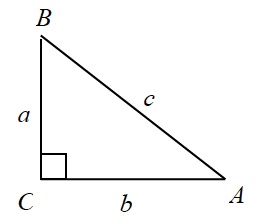Using the triangle on the right, find: Homework Help ✎

1. sin A

2. sec A

3. csc B

4. tan B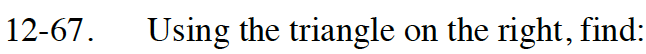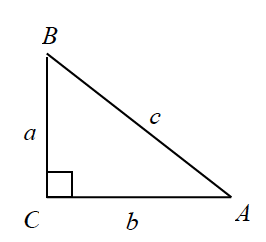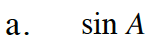Remember that sin(x) is opposite over hypotenuse.

$\sin \left(A\right)\ =\ \frac{a}{c}$

How is sec(x) related to cos(x)?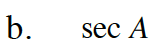Which trigonometric ratio is csc(x) related to? How is it related?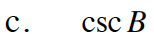Remember that tan(x) is opposite over adjacent.